Courses

# Test: Average- 4

## 15 Questions MCQ Test Quantitative Aptitude (Quant) | Test: Average- 4

Description
This mock test of Test: Average- 4 for Quant helps you for every Quant entrance exam. This contains 15 Multiple Choice Questions for Quant Test: Average- 4 (mcq) to study with solutions a complete question bank. The solved questions answers in this Test: Average- 4 quiz give you a good mix of easy questions and tough questions. Quant students definitely take this Test: Average- 4 exercise for a better result in the exam. You can find other Test: Average- 4 extra questions, long questions & short questions for Quant on EduRev as well by searching above.
QUESTION: 1

### Brian Lara, the famous batsman, scored 6,000 runs in certain number of innings. In the next five innings he was out of form and hence, could make only a total of 90 runs, as a result of which his average fell by 2 runs. How many innings did he play in all, if he gets out in all the innings?

Solution: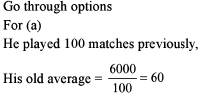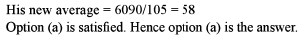QUESTION: 2

### The average weight of the books carried by Kuku to school is 1.5 kg and 2.5 kg is the average weight of the exercise books. If Kuku is carrying only text books and exercise books in the ratio of 3:4, what is the total weight of his school bag? (Ignore any other weights such as that of school bag, stationary etc.)

Solution:

No data regarding the numbers of books is given, hence we cannot determine the weight of school bag. Hence, (d)

QUESTION: 3

### The average of eight numbers is 25, that of the first two is 20 and of the next three is 26. The sixth number is less than the seventh by 4, and less than the eighth by 6. The last number is

Solution:

40 + 78 + x - 6 + x - 2 + x - 200

3x = 90

x = 30

QUESTION: 4

Three years ago , the average age of a family of 5 members was 17 years. Inspite of the birth of a child in the family, the present average age of the family remains the same. The present age of the child is

Solution:

Total age of family 3 years ago = 17x5 = 85 years

Total age of family now = 17x 6 = 102 years

Total age of family excluding the child now = (85 + 15) = 100 years

Age of child = 2 years

QUESTION: 5

The average marks of a class of 48 students is 35. Of them, two score zero, of the rest, the first 30 scored an average of 40, the next fourteen scored an average of 20. If the remaining two scored equal marks, what are their individual marks?

Solution: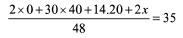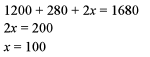QUESTION: 6

The average age of 2/3rd of the class is 17. What should be the average the age of the remaining l/3rd students so that the average age of the entire class is 20?

Solution: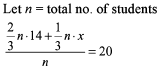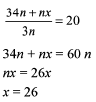QUESTION: 7

The average mark of a class of n students is 64. When eight new students with an average mark of 73 join the class, the new average of the entire class is a whole number. Find the number of students now in the class, given that n lies between 25 and 60.

Solution:

Let ‘x’ be the increase in the average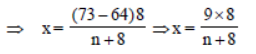For ‘x’ to be a whole number 72 (= 9 × 8) should be divisible by (n + 8)
From the choices it can be said that 36 and 72 are two such factors. But 72 does not lie within the range.
∴ number of students in class are 36.

QUESTION: 8

The average of 10 two-digit positive integers is Z. However, one number AB is taken as BA, then the average increases to Z + 2.7. What is the value of |B - A|?

Solution: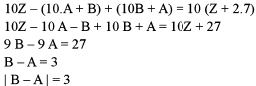QUESTION: 9

The average rainfall for the first 3 days out of five days was recorded to be 0.45 inches. The rainfall on the last two days was in the ratio 2:3. The average of five days was 0.40 inches. What was the rainfall on the last day?

Solution: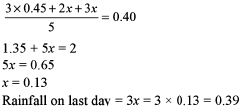QUESTION: 10

The average price of a share is the average of 5 readings taken at regular intervals in a day. The index price is taken by a weighted arithmetic average price of a class A and class B stock. The respective weights are 1.1 and 0.9 for the two kinds of stocks. If the five readings of a class A stock were 19, 26, 31, 35, 39 and for a class B stock the readings were 7, 8, 17, 20, 23 then what was the index price that day?

Solution: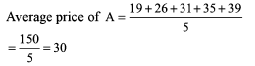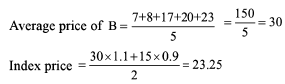QUESTION: 11

The average age of 24 students and the class teacher is 16 years. If the age of the class teacher is excluded the average reduces by 1 year. What is the age of the class teacher?

Solution: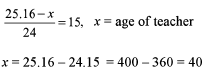QUESTION: 12

There are 20 students in Mr Rahul Ghosh’s class. He conducts an examination out of 100 and then arranged the marks in an ascending order. He found Chandan, the topper of the class, had slipped to the tenth position. When he was adding the scores of the last 11 students the average was 64 and that of the top 10 was 67. If the average marks obtained by all the students of his class was 65, how many marks did Chandan score?

Solution:

Score of Chandan = 64 x 11 + 10 x 67 - 20 x 65 = 704 + 670 - 1300
= 1374 -1300 = 74

QUESTION: 13

60 litres of a 75% solution of wine is taken into a laboratory. 4 litres of the solution is flushed and 4 litres of water is added to compensate the loss. Then 5 litres of the solution is flushed again and 5 litres of water is added. Again 6 litres of the solution is flushed and 6 litres of water is added. The final concentration of wine in the solution is

Solution:

Initial Concentration of wine = 75% Concentration of wine after 1st flushing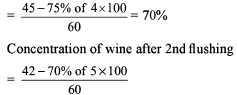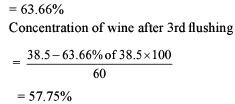QUESTION: 14

A vessel contains a mixture of two liquids A and B in the ratio of 4:1. When 10 litres of the mixture is replaced with liquid B, the ratio becomes 2:3. How many litres of liquid A was present in the jar initially?

Solution: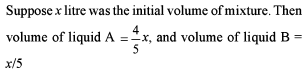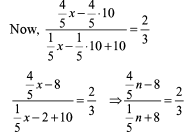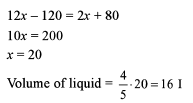QUESTION: 15

A vessel contains a mixture of two liquids A and B in the ratio of 7:5. When 9 litres of the mixture is taken out and the vessel is filled with B, the ratio of A and B is now 7:9. How many litres of liquid A was there in the vessel initially?

Solution:

Let 7x and 5x volume of liquids A and Liquid B are present in vessel then,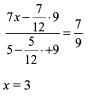Hence, 7.3 = 211 liquid A was present.